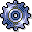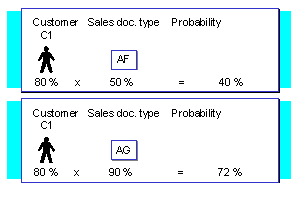Order ProbabilityUse

When you create a sales query, you may want to know: What is the probability that the customer will buy from me? The system calculates the probability from master data that can be defined for the sales document type and for specific customers. The estimate is expressed as a percentage. Based on the resulting probability, the system calculates an expected order value and displays it in the top section of the double-line entry overview screen. You can then generate a list of quotations or inquiries in such a way that sales queries with the highest probability of being confirmed are listed first. The following figure shows how the system calculates order probability from master data.